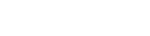# Conditional Statements

Conditional Statements allow us to control the flow of our program execution. If the code statements only executed one after the other until the end of a function, the functionality would be very limited.

Once upon a time, flow-charts were popular (maybe they still are?) where we could design our program flow with decision nodes to decide which branch to go down. Typically, we would test a condition for true or false and branch accordingly.

In a game, we are likely to have simple true/false conditions to test or, a more complex state with several possibilities.

The basic conditional statement uses the if / else / elif syntax. We use indentation to define the levels for how deep into the if structure we are. Here are some GDScript code examples:

``````extends Node2D

var n = 6

# Inline 'if' statement
if n == 6: print("n is equal to six")

n = 4
# Regular 'if' statement
if n == 4:
print("n is equal to four")

# 'else/if' statement
if n == 6:
print("n is equal to six")
else:
print("n is not equal to six")

# Messy indented 'else/if' statement
if n == 6:
print("n is equal to six")
else:
if n < 6:
print("n is less than six")
else:
print("n is greater than six")

n = 8
# Tidier 'else/if' statement using 'elif'
if n == 6:
print("n is equal to six")
elif n < 6:
print("n is less than six")
else:
print("n is greater than six")

``````

In the above code, you can see how indentation can get messy where there is more than one if test, so elif may be used to make the code tidier.

## Match Statement

In other programming languages, the switch statement is commonly provided to allow for conditional branching based on multiple cases of the test value. GDScript provides a powerful alternative Match statement.

Our game state is likely to be in one of many states which may be evaluated by a Match statement to decide which code needs to be executed to process the current game play.

## Ternary-if Expressions

This is a handy one-liner to assign a value to a variable based on a condition.

`var x = [value] if [expression] else [value]`

Code example:

``````var paid = false
var strength = 9.9 if paid else 1.0
print("Strength = ", strength)
``````

The next topic is GDScript Looping